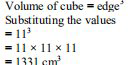# ML Aggarwal Solutions Class 9 Mathematics Solutions for Mensuration Exercise 16.4 in Chapter 16 - Mensuration

A cube of 11 cm edge is immersed completely in a rectangular vessel containing water. If the

dimensions of the base of the vessel are 15 cm × 12 cm, find the rise in the water level in centimeters correct

to 2 decimal places, assuming that no water overflows.

It is given that

Edge of cube = 11 cmWe know that

The dimensions of the base of the vessel are 15 cm × 12 cm

Consider the rise in the water level = h cm

So the volume of cube = volume of the vessel

Substituting the values

1331 = 15 × 12 × h

By further calculation

h = 1331/ (15 × 12)

So we get

h = 1331/ 180 = 7.39 cm

Therefore, the rise in the water level is 7.39 cm.

Video transcript
"hello and welcome idea students i am rishabh the math to the run leader and i'm here with a new question it is a cube of 11 centimeter edge is immersed completely in a rectangular vessel containing water if the dimensions of the base of the vessel are 15 centimeter into 12 centimeter finally rise in the water level and the centimeters corrected two decimal places assuming that no water overflows so without wasting much time let's have a look at the solution so we have been given that the edge of cube is 11 centimeter we'll first find out the volume of the cube the volume of cube is nothing but the side cube which is 11q solving it we get one three three one centimeter q now we know that the dimensions of the vessel are 15 into 12. so we have been given the dimensions of the base of the vessel are 15 centimeter into 12 centimeter now let's consider consider the rise in the water level has h centimeter and we know that the volume of q is equal to volume of vessel so we'll substitute the values and we get 1 3 3 1 is equals to 15 into 12 into h which is nothing but equals to 1 3 3 1 divided by 180 so multiplying 15 and 12 will get 180 so dividing it we get 7.39 centi meters be careful that we are supposed to find out the answer up to two decimal places so the height is 7.39 centimeter i hope my students got this question well do not forget to hit the subscribe button you can also share your doubts in the comment section see you next time bye "
Related Questions

Lido

Courses

Teachers

Book a Demo with us

Syllabus

Maths
CBSE
Maths
ICSE
Science
CBSE

Science
ICSE
English
CBSE
English
ICSE
Coding

Terms & Policies

Selina Question Bank

Maths
Physics
Biology

Allied Question Bank

Chemistry
Connect with us on social media!